# 12 Atomic Experiments in Deep Learning¶

Deep Learning has been compared to electricity. I'd like to provide another analogy: alchemy. -- Ali Rahimi, NIPS 2017

• Deep learning remains somewhat of a mysterious art even for frequent practitioners, because we usually run complex experiments on large datasets, which obscures basic relationships between dataset, hyperparameters, and performance.
• The goal of this notebook is to provide some basic intuition of deep neural networks by running very simple experiments on small datasets that help understand trends that occur generally on larger datasets. The experiments are designed to be "atomic" in that they try to test one fundamental aspect of deep learning in a controlled way. Furthermore, the experiments do not require specialized hardware (they all run in a few minutes without GPUs. See the elapsed time, measured on a CPU-only machine).
• All of the experiments here are written in Python using the tensorflow and sklearn libraries, but most of the code has been abstracted away to make the experiments easier to run. See the Github repo for full code.

# I. Basics¶

## 1. Why Use Neural Networks in the First Place?¶

The universal approximation theorem, states that a simple feedforward neural network (i.e. a multilayer perceptron) with a single hidden layer and a standard activation function can approximate any continuous function, if the hidden layer has a sufficient number of units. Let's show this in practice by seeing how many units it takes to approximate some select functions.

Method: We'll train a 1-layer neural network on 50 datapoints $(x,y)$ that are drawn from the following functions on the domain $[-1,1]$, and measure the mean square error (MSE) of the resulting fit. We'll try out the following functions (feel free to try your own by changing the code below!):

• $f(x) = \sin(12x)$
• $g(x) = -2x^4 + x^3 + x^2 - 2x + 0$
• $h(x) = \lvert x \rvert$

Hypothesis: As the number of units in the hidden layer increases, the accuracy of the resulting fit will increase (error will decrease).

In :
functions = [sin(omega=12),
polynomial([0,-2,1,1,-2]),
np.abs]
n_hidden_units = [2,4,16]
experiment = Experiment1()

f, axs = plt.subplots(4,3,figsize=(12,15))
plt.rc("font",family="sans-serif",size=14)

for i, n_hidden in enumerate(n_hidden_units):
ax = axs[0, i]
ax.axis('off')
draw_neural_net(ax, .15, .9, .1, .85, [1, n_hidden, 1])

experiment.initialize()
plot_idx = 1
for i, function in enumerate(functions):
for j, n_hidden in enumerate(n_hidden_units):
x_values, y_values, y_pred, loss = experiment.run(n_hidden=n_hidden, function=function, verbose=False)
ax = axs[i+1, j]
ax.plot(x_values, y_values, '-',label='True y')
ax.plot(x_values, y_pred, '--',label='Predicted y')
ax.set_xlabel('x')
ax.set_ylabel('y')
if (j==0):
ax.legend()
ax.set_title(str(n_hidden)+' hidden units, MSE='+str(np.round(loss,4)))

plt.tight_layout()
experiment.conclude()

Time needed to run experiment: 91.595 s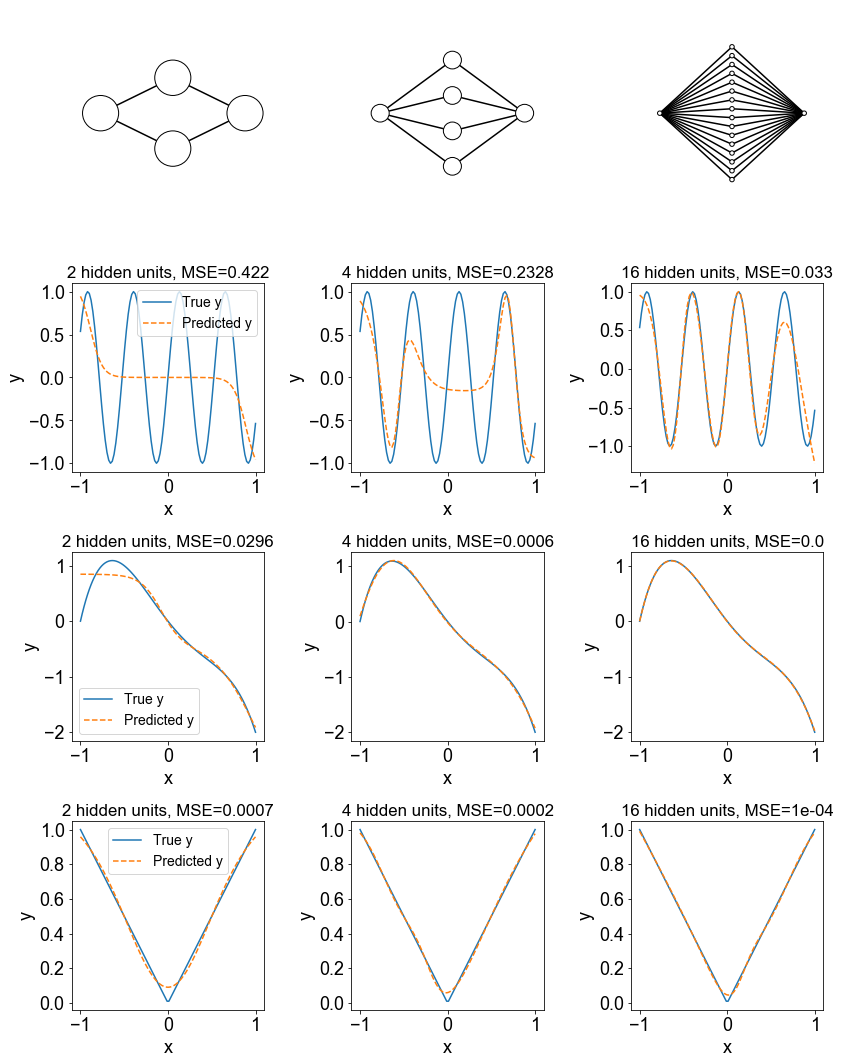Conclusion: As the number of hidden units increase, the approximation error on the training data generally decreases.

Discussion: Although the universal approximation theorem says that neural network with enough parameters can approximate a true classification/regression function, it doesn't say anything about whether these parameters are learnable through a procedure like stochastic gradient descent. Additionally, you might be wondering whether we can theoretically calculate how many neurons are needed to approximate a given function arbitrarily well. See (Mhaskar, 1996) for some discussion of this.

## 2. Why are Deeper Networks Better?¶

In practice, deeper multilayer perceptrons (with more than 1 hidden layer) outperform shallow ones by a wide margin on many task of interest. Why might this be the case? It has been suggested that deeper neural networks require fewer parameters to express many important classes of functions. It has been shown theoretically that expressing simple radial functions and compositional functions requires an exponentially large number of parameters using shallow networks, but not so for deep neural networks. Spoiler alert: I tried to experimentally verify these papers, but I was not able to do so (this does not invalidate the results of the paper -- just because a set of neural network parameters exist, does not mean they can be learned easily by stochastic gradient descent). The only result I was able to reproduce even somewhat reliably was from a paper that proposed a family of difficult classification problems that are easier for deeper neural networks. This is what I show here.

Method: The dataset consists of 16 equally-spaced points along the x-axis, with each pair of ajacent points belonging to opposite classes. A special kind of deep neural network (one that shares weights across layers) with a fixed number (152) of parameters but varying numbers of layers is tested.

Hypothesis: As the number of layers increase in a neural network with a fixed number of parameters, the accuracy on a difficult classification problem will improve.

In :
experiment = Experiment2()
experiment.initialize()

n_hidden_units = [,[10,10],[10,10,10]]

f, axs = plt.subplots(2,3,figsize=(12,8))
plt.rc("font",family="sans-serif",size=14)

for i, n_hidden in enumerate(n_hidden_units):
ax = axs[0, i]
ax.axis('off')
draw_neural_net(ax, .15, .9, .1, .85,  + *len(n_hidden) + ) #10 hidden units per layer becomes too crowded

for i, n_hidden in enumerate(n_hidden_units):
ax = axs[1, i]
x_values,y_values,y_pred,loss,accuracy,n_params = experiment.run(n=16, n_hidden = n_hidden)
ax.set_title(str(len(n_hidden))+' layers, '+str(n_params)+' params, Acc: '+str(np.round(100*accuracy,1))+'%')
ax.set_xlabel('x')
ax.set_ylabel('True label')
ax.scatter(x_values, y_values[:,1], marker='o',c=y_values[:,1],cmap='bwr',label='Predicted y')
ax2 = ax.twinx()
ax2.plot(x_values, y_pred[:,1]-y_pred[:,0], 'k--o',label='Predicted y')
ax2.axhline(0, color='k', ls='-')
ax2.set_ylabel('Predicted label')

plt.tight_layout()
experiment.conclude()

Time needed to run experiment: 28.688 s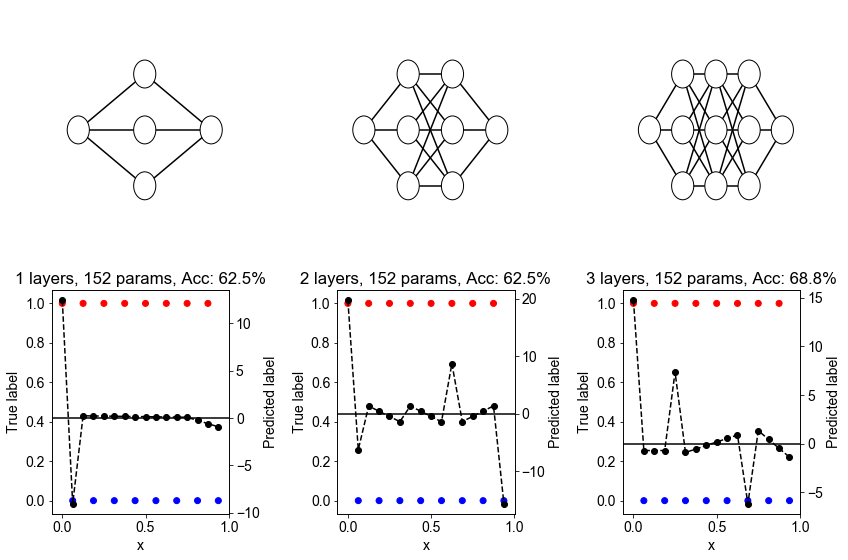Here, red and blue dots represent points that belong to different classes. The dashed-dotted black line is the closest approximation to the training data that is learned by the neural network (if the neural network assigns a score greater than zero, then a point is considered to be predicted as belonging to the red class. Otherwise, it is predicted to be blue). The zero line is shown in black.

Conclusion: Accuracy increases with increasing depth in most runs of the experiments.

Discussion: It seems that deeper layers allow more "sharp turns" in the learned function from the input to the output. This seems to be related to some work on the trajectory length of neural networks (a measure of how much the output of a neural network travels as the input varies along a 1D path of fixed length).

# II. Datasets¶

## 3. Does More Data Favor Deeper Neural Networks?¶

Deep learning and big data are closely linked; it's usually said that deep learning only works better than other techniques (such as shallow neural networks and random forests) when the size of the dataset grows large enough to overcome overfitting, and favor the increased expressivity of deep networks. We investigate this on a very simple dataset, consisting of a mixture of Gaussian samples.

Method: The dataset consists of a mixture of two 12-dimensional Gaussians, each of which generate data belonging to one class. The two Gaussians have the same covariance matrix, but have means that are $1/i$ unit apart in the $i^\text{th}$ dimension. The idea is to have some dimensions that allow models to easily discriminate between classes, while other dimensions are more difficult, but still useful, for discrimination.

Hypothesis: As the size of dataset increases, the test accuracy of all of the techniques will improve, but the accuracy of deep models will grow more than the non-deep models. We further expect the accuracy of the non-deep learning techniques to saturate more quickly.

In :
from sklearn.neural_network import MLPClassifier
from sklearn.tree import DecisionTreeClassifier
from sklearn.naive_bayes import GaussianNB
from sklearn.svm import SVC

ns = [20, 50, 100, 200, 500, 1000, 2000, 5000, 10000]
test_size=0.2; train_size=1-test_size

class_seps = [1/(i+1) for i in range(12)]

classifiers = [SVC(),
DecisionTreeClassifier(),
MLPClassifier(),
MLPClassifier(hidden_layer_sizes=(100,100)),
]

classifier_names = ['Support Vector Machine',
'Decision Trees',
'Shallow Network',
'Deep Network']

experiment = Experiment3()
experiment.initialize()

plt.figure(figsize=[8,8]); plt.rc("font",family="sans-serif",size=18)
accuracies = experiment.run(ns=ns,classifiers=classifiers,class_seps=class_seps,d=12,iters=3, test_size=test_size)
plt.title('Test Accuracy of Various Classifiers')
plt.plot(train_size*np.array(ns), np.mean(accuracies,axis=2), '-o')
plt.xlabel('Training set size (n)')
plt.ylabel('Test Accuracy')
plt.xscale('log')
plt.legend(classifier_names)

experiment.conclude()

Time needed to run experiment: 138.239 s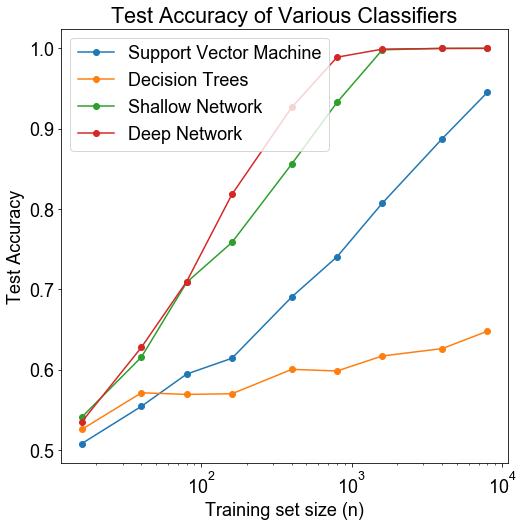Conclusion: The neural networks consistently perform better than SVMs and random forests across dataset size. The gap in performance increases as dataset size increases in, at least until the accuracy of the neural networks begin to saturate, suggesting that the neural networks are more efficiently able to take advantage of increasing dataset size. However, with enough data, even SVMs have a respectable accuracy. Deep networks perform a little bit better than shallow networks.

Discussion: Although increasing dataset size does tend to favor neural networks as we expected, it is interesting that the neural networks are already better than other techniques on relatively small datasets. It seems that the 2-layer network isn't significantly overfitting, even though we would expect some features (say features 6-12, which have low levels of signal) to cause the networks to overfit. It's also interesting that the SVM seems like it could have an approaching 1.0 with enough data.

## 4. Does Unbalanced Data Hurt Neural Networks?¶

When datasets are unbalanced (if there are many more examples of one class than another), then neural networks may not be able to learn how to distinguish the classes. In this experiment, we explore whether this is the case, and we also explore whether oversampling, a popular remedy in which samples from the minority class are sampled with replacement, can mitigate the probelm.

Method : We generate a mixture of two 2-dimensional (results not shown here indicated that the same results hold for higher dimensions) Gaussians, each of which generate data belonging to one class. The two Gaussians have the same covariance matrix, but have means that are $1/i$ unit apart in the $i^\text{th}$ dimension. Each training dataset consists of 1,200 data points, but we vary the class imbalance from 1:1 to 1:99. The test dataset remains fixed at a 1:1 ratio to make it easy to compare performance, and consists of 300 points. We also show the decision boundary in each case.

Hypothesis : We expect test accuracy to decrease with increasing class imbalance, but we expect oversampling to mitigate this problems.

In :
ratios = [1, 2, 5, 10, 20, 50, 100]
experiment = Experiment4()
experiment.initialize()

plt.figure(figsize=[8,6]); plt.rc("font",family="sans-serif",size=18)

accuracies, saved_covs = experiment.run(ratios=ratios,
d=2,
iters=5,
resample=False)
plt.plot(ratios, np.mean(accuracies,axis=1), '-o')
plt.fill_between(ratios, np.mean(accuracies,axis=1)-np.std(accuracies,axis=1),np.mean(accuracies,axis=1)+np.std(accuracies,axis=1), alpha=0.3)

accuracies, _ = experiment.run(ratios=ratios,
d=2,
iters=5,
resample=True,

plt.plot(ratios, np.mean(accuracies,axis=1), '-o')
plt.fill_between(ratios, np.mean(accuracies,axis=1)-np.std(accuracies,axis=1),np.mean(accuracies,axis=1)+np.std(accuracies,axis=1), alpha=0.3)

plt.title('Accuracy on Imbalanced Datasets')
plt.xlabel('Ratio (X:1)')
plt.ylabel('Test Accuracy')
plt.xscale('log')
plt.legend(['Without resampling','With resampling'])

plt.figure(figsize=[10,5]); plt.rc("font",family="sans-serif",size=18)

accuracies, saved_covs, X_train, y_train, X_test, y_test, y_pred, grid_pred = experiment.run(ratios=,
d=2,
iters=1,
resample=False,
classify_grid=True)

plt.subplot(1,2,1)
plot_decision_boundary(X_train, y_train, grid_pred)
plt.title('Training, no resampling')
plt.subplot(1,2,2)
plot_decision_boundary(X_test, y_test, grid_pred)
plt.title('Test, no resampling, acc='+str(round(accuracies,2)))
plt.tight_layout()

plt.figure(figsize=[10,5]); plt.rc("font",family="sans-serif",size=18)

accuracies, _, X_train, y_train, X_test, y_test, y_pred, grid_pred = experiment.run(ratios=,
d=2,
iters=1,
resample=True,
classify_grid=True,

plt.subplot(1,2,1)
plot_decision_boundary(X_train, y_train, grid_pred)
plt.title('Training, resampling')
plt.subplot(1,2,2)
plot_decision_boundary(X_test, y_test, grid_pred)
plt.title('Test, resampling, acc='+str(round(accuracies,2)))
plt.tight_layout()

experiment.conclude()

Time needed to run experiment: 392.157 s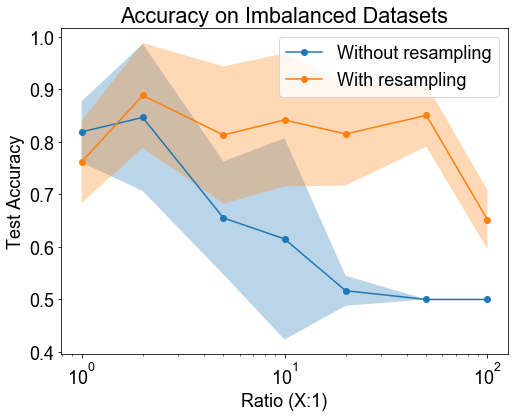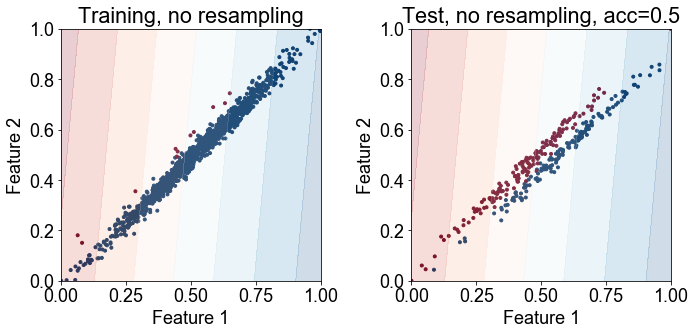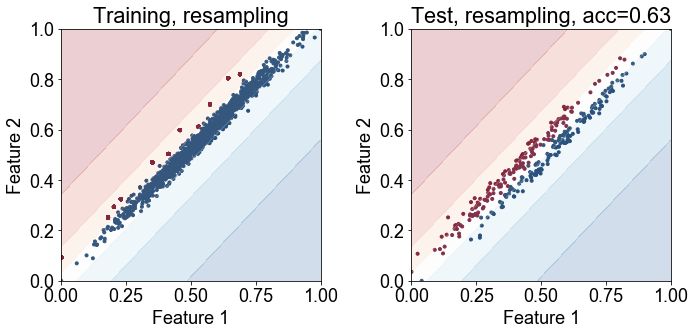The bottom four plots show countours of the decision boundary plotted along with the training points (left) or test points (right). The top row shows the result without resampling and the bottom with resampling.

Conclusion: The results show that class imblaance certainly does reduce classification accuracy. Resampling can significantly improve the performance..

Discussion: It is perhaps surprising that resampling has a significant effect in improving classification accuracy, since it does not expose the classifier to new training examples from the minority class. But the plots suggest that resampling is enough "nudge" or rotate the decision boundary in the right direction. In cases where resampling is not effective alone, complex methods to synthesize new training examples from the minority class may be needed to improve accuracy.

## 5. How Do You Tell if a Neural Networks is Memorizing Or Generalizing?¶

Neural networks with many parameters have the capacity to memorize lots of training examples. So are neural networks simply memorizing the training examples (and then simply classifying test points based on the closest similar training point), or are they actually picking up patterns and generalizing? Is there any difference? One reason to think there is a difference is if the speed at which a neural network learns randomly-assigned labels is different than it which it learns reaon labels. This is one of strategies used in the paper by Arpit et al.. Let's see if we see a difference.

Method: First, we generate a mixture of 6-dimensional Gaussians and randomly assign them labels. We measure the accuracy on the training data for increasing data set sizes to get a sense of the capacity of a neural network to memorize. We then choose a dataset size within the capacity of a neural network to memorize and observe whether there are qualitative differences between a neural network during training that is learning random labels versus real labels. In particular, we look at the accuracy per epoch to determine whether neural networks that are learning true labels or random labels.

Hypothesis: We expect that training should take longer for random labels as opposed to real labels.

In :
ns = [50, 100, 150, 200, 250, 300]
experiment = Experiment5()
experiment.initialize()

plt.rc("font",family="sans-serif",size=18)
fig, (ax1, ax2) = plt.subplots(1, 2, figsize=[12,6])

accuracies = experiment.run(ns=ns,hidden_layer_sizes=[200,200,200], return_accuracy_per_epoch=True)
ax1.plot(ns, np.mean(accuracies[-1],axis=1), '-o')
ax1.fill_between(ns, np.mean(accuracies[-1],axis=1)-np.std(accuracies[-1],axis=1),np.mean(accuracies[-1],axis=1)+np.std(accuracies[-1],axis=1), alpha=0.3)
ax1.set_ylim([0,1.04])

steps = np.linspace(250,2500,10)
ax2.plot(steps, np.mean(accuracies[:,2,:],axis=1), '-o')
ax2.fill_between(steps, np.mean(accuracies[:,2,:],axis=1)-np.std(accuracies[:,2,:],axis=1),np.mean(accuracies[:,2,:],axis=1)+np.std(accuracies[:,2,:],axis=1), alpha=0.3)
ax2.set_ylim([0,1.04])

accuracies = experiment.run(ns=ns,hidden_layer_sizes=[200,200,200], randomize=True, return_accuracy_per_epoch=True)
ax1.plot(ns, np.mean(accuracies[-1],axis=1), '-o')
ax1.fill_between(ns, np.mean(accuracies[-1],axis=1)-np.std(accuracies[-1],axis=1),np.mean(accuracies[-1],axis=1)+np.std(accuracies[-1],axis=1), alpha=0.3)
ax1.legend(['True labels', 'Random labels'])
ax1.set_xlabel('n')
ax1.set_ylabel('Training Accuracy')
ax1.set_title('Neural Network Memorization')

ax2.plot(steps, np.mean(accuracies[:,2,:],axis=1), '-o')
ax2.fill_between(steps, np.mean(accuracies[:,2,:],axis=1)-np.std(accuracies[:,2,:],axis=1),np.mean(accuracies[:,2,:],axis=1)+np.std(accuracies[:,2,:],axis=1), alpha=0.3)
ax2.legend(['True labels', 'Random labels'])
ax2.set_xlabel('Epoch')
ax2.set_ylabel('Training Accuracy')
ax2.set_title('Training Accuracy (n=150)')

experiment.conclude()

Time needed to run experiment: 432.275 s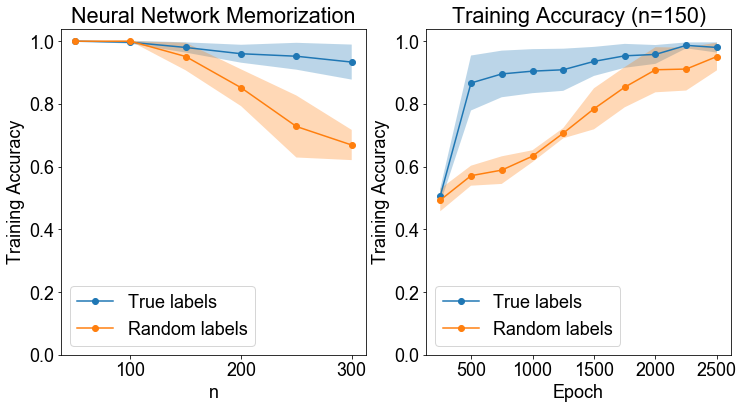Conclusion: The capacity of the neural network to memorize seems to be about 150 training points. But, even then, the neural network takes longer to learn random labels as opposed to ground truth labels.

Discussion: This result is not very surprising. We would expect true labels to be learned more quickly -- when a neural network learns to correctly classify one particular data point, it will also learn to classify other similar data points -- if the labels are sensical, but not if they are random!

## 6. Does Unsupervised Dimensionality Reduction Help or Hurt?¶

When dealing with very high dimensional data, it may be difficult for a neural network to learn the correct classification boundary. In these situations, one may consider doing unsupervised dimensionality reduction before passing the data into the neural network. Does this help or hurt?

Method: We generate a mixture of two 10-dimensional Gaussians. The Gaussians have the same covariance matrix, but have means that are separated by 1 in every dimension. We then add to the data "dummy dimensions" which are features that take very low random values for both types of Gaussians, and are thus not useful for classification. We then apply multiply the resulting data by a random rotation matrix to obfuscate the dummy dimensions. The small dataset size ($n=100$) makes it difficult for a neural network to learn the classification boundary. So we PCA the data into a smaller number of dimensions and see if the classification accuracy increases.

Hypothesis: We expect that PCA will help since the directions with the most variation also (likely) correspond to the directions that are the most useful for classification.

In :
experiment = Experiment6()
experiment.initialize()

dummy_dims = [1, 2, 5, 10, 20, 50, 100, 200, 500, 1000, 2000]
pca_dims = [None, 10, 5, 1]

accuracies = experiment.run(dummy_dims=dummy_dims,
noise_level=1,
pca_dims=pca_dims)

plt.figure(figsize=[8,8]); plt.rc("font",family="sans-serif",size=18)
plt.plot(dummy_dims, np.mean(accuracies,axis=0), '--o')

plt.title('Test Accuracy with Unsupervised Preprocessing')
plt.xlabel('Dummy Dimensionality')
plt.ylabel('Test Accuracy')
plt.xscale('log')
plt.legend(['No PCA', 'PCA dim = 10', 'PCA dim = 5', 'PCA dim = 1'])

experiment.conclude()

Time needed to run experiment: 182.938 s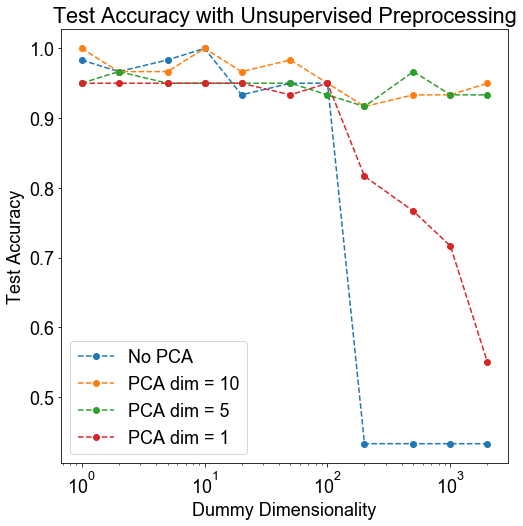Conclusion: An unsupervised PCA step can provide significant improvement in downstream classification when the dimensionality is very large.

Discussion: We observe an interesting thresholding behavior. When the dimensionality increases beyond 100 (which, interestingly, is the number of data points in the dataset -- this should be explored further), the quality of classification drops significantly. In these cases, PCA to 5 or 10 dimensions significantly improves downstream classification

# III. Parameters and Hyperparameters¶

## 7. Can Any Non-Linearity be Used as the Activation Function?¶

Many research papers have been published on marginal improvements obtained by neural networks with special activation functions beyond the typical $\text{ReLU}(\cdot)$'s and $\tanh(\cdot)$. Instead of trying to develop specialized activation functions, we simply ask whether it is possibly to use any old non-linear function in a neural network?

Method: We generate the famous two-dimensional moons dataset, and train a neural network with 2 hidden layers to learn to classify the dataset. We try 7 different activation functions:

• $f(x) = \text{ReLU}(x)$
• $f(x) = \tanh(x)$
• $f(x) = x^2$
• $f(x) = \sin(x)$
• $f(x) = \text{sign}(x)$
• $f(x) = x$

Hypothesis: We would expect that the identity function to perform poorly (as the network remains quite linear until the last softmax layer). We might further expect that the standard activation functions to perform the best.

In :
experiment = Experiment7()
experiment.initialize()

activations = [tf.nn.relu, tf.tanh, tf.square, tf.sin, tf.sign, tf.identity]
x = np.linspace(-10,10,1000)

accuracies = experiment.run(activations=activations,
iters=3)

fig, axs = plt.subplots(1, 6, figsize=[15,3])
sess = tf.Session()
for i, ax in enumerate(axs):
ax.plot(x, sess.run(activations[i](x)))
ax.set_title(activations[i].__name__)
plt.tight_layout()

plt.figure(figsize=[8,8]); plt.rc("font",family="sans-serif",size=18)
plt.plot(np.mean(accuracies,axis=0), '--o')

plt.title('Test Accuracy with Various Activations')
plt.xlabel(r'$\propto$ Epochs')
plt.ylabel('Test Accuracy')
plt.legend([a.__name__ for a in activations])

experiment.conclude()

Time needed to run experiment: 22.745 s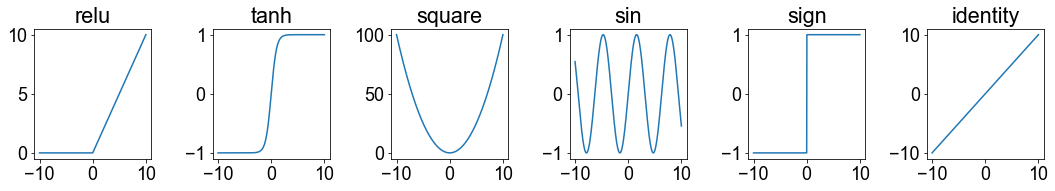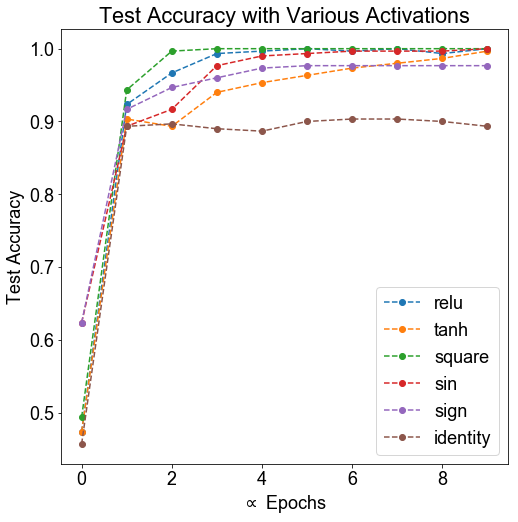Conclusion: All of the non-linear activation functions besides $\text{sign}(x)$ are quite effective for this classification task.

Discussion: The results are somewhat surprising since all of the functions are about equally effective. In fact, symmetric activation functions, like $x^2$ seem to be performing as well as $\text{ReLUs}$! There are a couple of reasons we should be careful about extrapolating too much from this experiment. For one, this is a relatively shallow neural network. Activation functions that are good for this kind of network may be very different than those that are good for deeper networks. Furthermore, this task may have been too easy -- as even the almost completely linear neural network ($f(x) = x$) achieves about 88% accuracy!

## 8. How Does Batch Size Affect Test Accuracy?¶

Method: We generate a mixture of two 12-dimensional Gaussians. The Gaussians have the same covariance matrix, but have means that are separated by 1 in every dimension. The dataset consists of 500 Gaussians, of which 400 are used to train, and 100 used to test. We train a neural network on this dataset with batches of different sizes from a batch size of 1 to a batch size of 400. We measure both the accuracy after

Hypothesis: We expect that larger batch sizes will increase test accuracy (less noisy gradient updates), up to a certain extent, after which the test accuracy will decrease. We expect the running time to go down with increasing batch size.

In :
experiment = Experiment8()
experiment.initialize()

batch_sizes = [1, 4, 16, 64, 256, 400]
runtimes, accuracies = experiment.run(iters=4,
batch_sizes=batch_sizes,
return_accuracy_per_epoch=True)

acc_thres = 0.8
time_to_thres = np.multiply(runtimes/10,np.argmax(np.mean(accuracies,axis=2)>acc_thres,axis=0))

plt.figure(figsize=[12,6]); plt.rc("font",family="sans-serif",size=18)
plt.subplot(1,2,1)
plt.plot(np.mean(accuracies,axis=2), '--o')

plt.title('Test Accuracy')
plt.xlabel('Epoch (x10)')
plt.ylabel('Test Accuracy')
plt.legend(['Batch of ' + str(b) for b in batch_sizes])

ax1 = plt.subplot(1,2,2)
ax1.plot(batch_sizes, runtimes, 'k-o')
ax1.set_title('Run time')
ax1.set_ylabel('Time (seconds)')
ax1.legend(['Runtime (total)'], loc='center right')

ax2 = ax1.twinx()
ax2.plot(batch_sizes, time_to_thres, 'r-o')
ax2.legend(['Time until acc='+str(acc_thres)])
ax2.set_xlabel('Batch Size')
ax2.set_ylabel('Time (seconds)')

plt.tight_layout()

experiment.conclude()

Time needed to run experiment: 293.145 s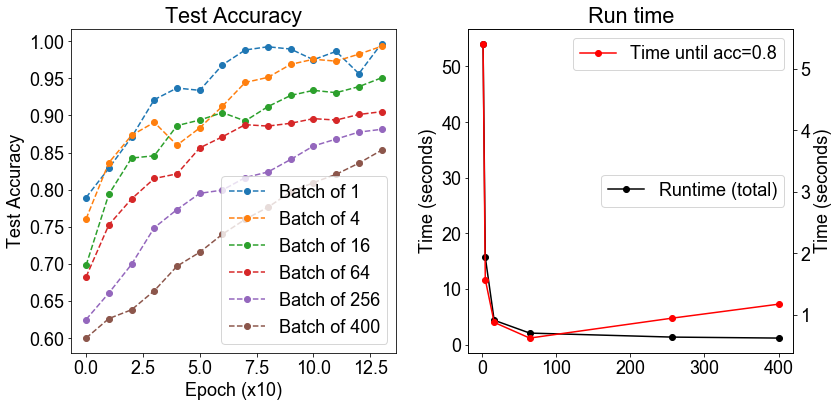Conclusion: The running time did decrease with increasing batch size, as we expected. However, this led to a compromise in test accuracy, as the test accuracy monotonically decreased with increasing batch size.

Discussion: This is pretty interesting, and is not in agreement with the widespread idea that moderately large batch sizes are strictly better for training. This may be due to the fact that we did not adjust the learning rate for different batch sizes. Because larger batch sizes do run faster, overall, the best compromise for batch size seems to be about a batch size of 64.

## 9. Does the Loss Function Matter?¶

For classification tasks, the cross-entropy loss is commonly used. What if we use mean-squared error instead, as is usually done on regression tasks? Does it matter which one we choose?

Method: We generate a mixture of two 12-dimensional Gaussians. The Gaussians have the same covariance matrix, but have means that are separated by 1 in every dimension. The dataset consists of 500 Gaussians, of which 400 are used to train, and 100 used to test. We train a neural network on this dataset with several different loss functions to see whether there are systematic differences in the final accuracy. As a negative control, a constant loss is included.

Hypothesis: We expect the cross entropy loss, as the standard loss function used for classification tasks, to perform the best, while we expect the other loss funtions to not perform as well.

In :
experiment = Experiment9()
experiment.initialize()

loss_functions = ['cross_entropy',
'mean_abs_error',
'mean_squared_error',
'mean_fourth_pow_error',
'hinge_loss',
'constant']

accuracies = experiment.run(loss_functions=loss_functions,
iters=5)

plt.figure(figsize=[8,8]); plt.rc("font",family="sans-serif",size=18)
plt.plot(np.mean(accuracies,axis=0), '--o')

plt.title('Test Accuracy with Different Losses')
plt.xlabel(r'$\propto$ Epochs')
plt.ylabel('Test Accuracy')
plt.legend(loss_functions)

experiment.conclude()

Time needed to run experiment: 36.652 s